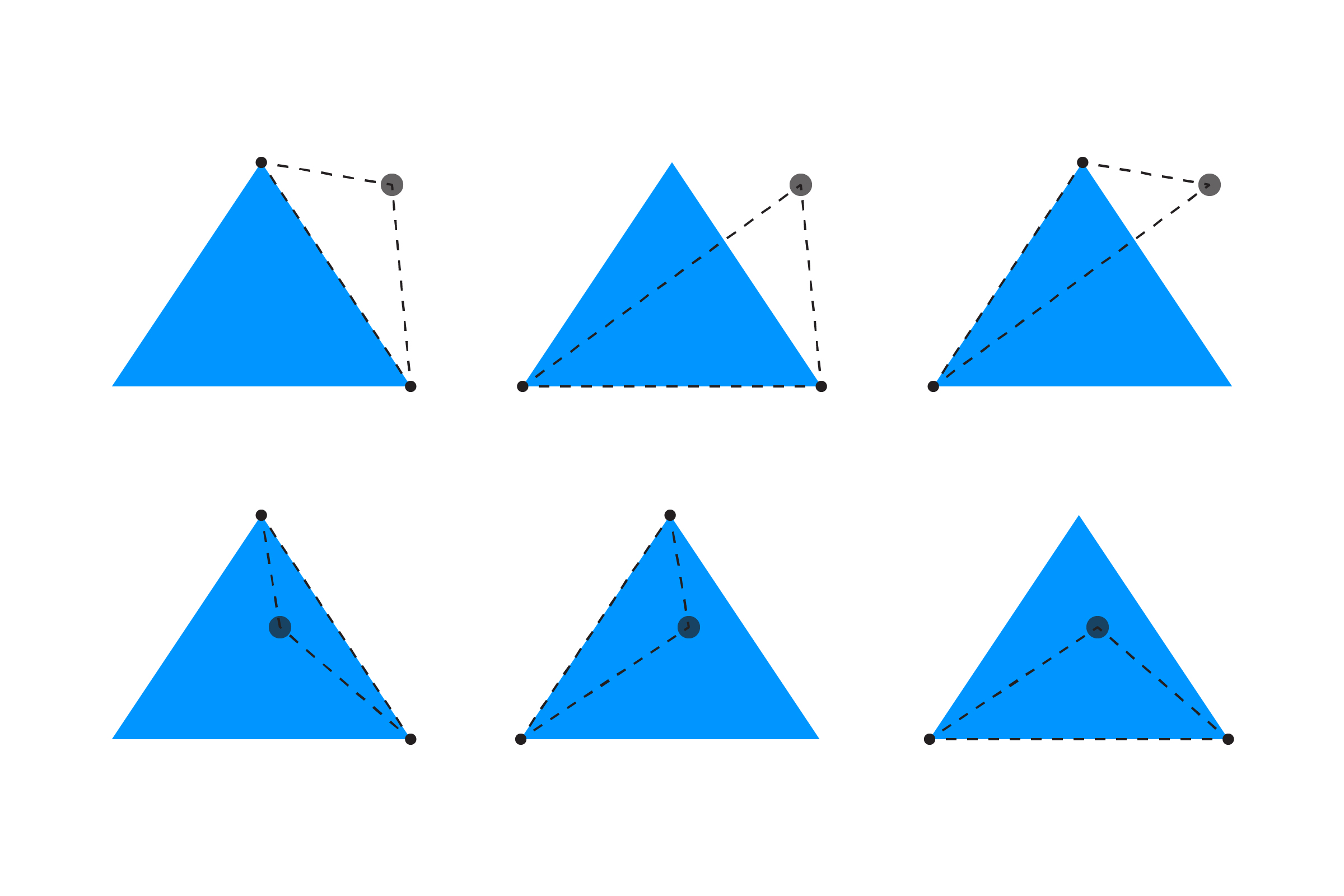[ your browser does not support the canvas tag ]

# TRIANGLE/POINT

To test if a point is inside a triangle, we compare the area of the original triangle with the sum of the area of three triangles made between the point and the corners of the triangle.

Here's a diagram demonstrating the triangles created for a point outside and inside the triangle:To get the area, we use Heron's Forumula:

``````float areaOrig = abs( (x2-x1)*(y3-y1) - (x3-x1)*(y2-y1) );
``````

We need to calculate the area of the three triangles made from the point as well:

``````float area1 =    abs( (x1-px)*(y2-py) - (x2-px)*(y1-py) );
float area2 =    abs( (x2-px)*(y3-py) - (x3-px)*(y2-py) );
float area3 =    abs( (x3-px)*(y1-py) - (x1-px)*(y3-py) );
``````

If we add the three areas together and they equal the original, we know we're inside the triangle! Using this, we can test for collision:

``````if (area1 + area2 + area3 == areaOrig) {
return true;
}
return false;
``````

Here's a full example:

``````float px = 0;        // point (set by mouse)
float py = 0;

float x1 = 300;      // three points of the triangle
float y1 = 100;
float x2 = 450;
float y2 = 300;
float x3 = 150;
float y3 = 300;

void setup() {
size(600,400);
noCursor();

strokeWeight(5);   // make point easier to see
}

void draw() {
background(255);

// mouse point to mouse coordinates
px = mouseX;
py = mouseY;

// check for collision
// if hit, change fill color
boolean hit = triPoint(x1,y1, x2,y2, x3,y3, px,py);
if (hit) fill(255,150,0);
else fill(0,150,255);
noStroke();
triangle(x1,y1, x2,y2, x3,y3);

// draw the point
stroke(0, 150);
point(px,py);
}

// TRIANGLE/POINT
boolean triPoint(float x1, float y1, float x2, float y2, float x3, float y3, float px, float py) {

// get the area of the triangle
float areaOrig = abs( (x2-x1)*(y3-y1) - (x3-x1)*(y2-y1) );

// get the area of 3 triangles made between the point
// and the corners of the triangle
float area1 =    abs( (x1-px)*(y2-py) - (x2-px)*(y1-py) );
float area2 =    abs( (x2-px)*(y3-py) - (x3-px)*(y2-py) );
float area3 =    abs( (x3-px)*(y1-py) - (x1-px)*(y3-py) );

// if the sum of the three areas equals the original,
// we're inside the triangle!
if (area1 + area2 + area3 == areaOrig) {
return true;
}
return false;
}
``````

This example was built on a modified version of a post on YoYo Games. If you would like to read a lengthy discussion on the merits and problems with this method, and many other suggestions, see this thread on GameDev.net.

NEXT: Where Are The Other Triangle Examples?